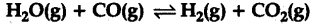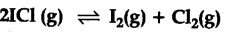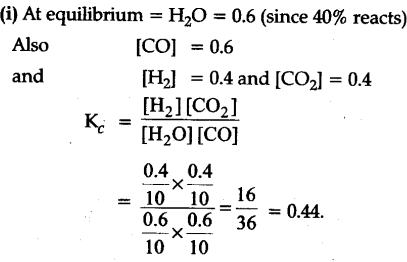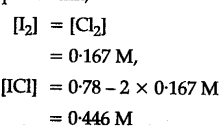# 1 mole of \${{H}_{2}}\$O and 1 mole of CO are taken in a 10 litre vessel and heated at 125 K. At equilibrium 40% of water (by mass) reacts with CO according to the equation

1 mole of \${{H}_{2}}\$O and 1 mole of CO are taken in a 10 litre vessel and heated at 125 K. At equilibrium 40% of water (by mass) reacts with CO according to the equation.Calculate equilibrium constant
(ii) What is the equilibrium concentration of each of the substances in the equilibrium when the initial concentration of ICI was 0.78 M ?